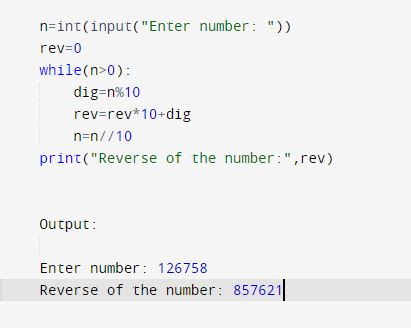# Python Program To Reverse the Given Number | RRTutors

Published January 07, 2021

In this program we will write a python program to revese the given number.

We can reverse a number in python by belwo ways

• Using for-loop
• Using while-loopUsing for-loop

 x=(input("Enter a Number: x= ")) revstr=[] ran = len(x)  for element in reversed(range(0, ran)):      revstr.append(x[element])      rev="".join(revstr)     print(rev)

Using while-loop

 n=int(input("Enter number: "))     rev=0     while(n>0):         dig=n%10         rev=rev*10+dig         n=n//10     print("Reverse of the number:",rev)

Output:

 Enter number: 126758 Reverse of the number: 857621

• Take input from user and store it in a variable n.
• By using the while loop we obtained last character by using modulus operator
• The last digit will stored at one’s place, next second is at  the ten’s place and so on.
• The last digit is then removed by truly dividing the number with 10.
• Then While loop will return when the value of the number is 0.
• Finally revere of the given number will return

 Article Contributed By :1264 Views
Projects By category
Categories